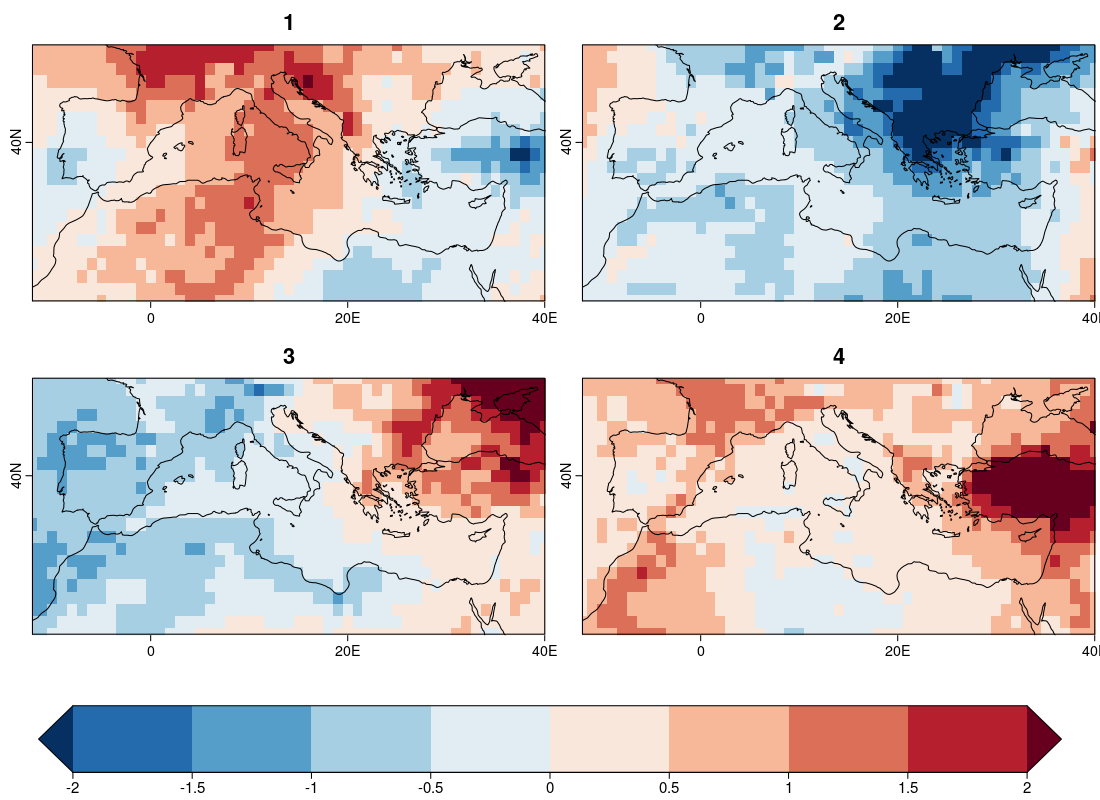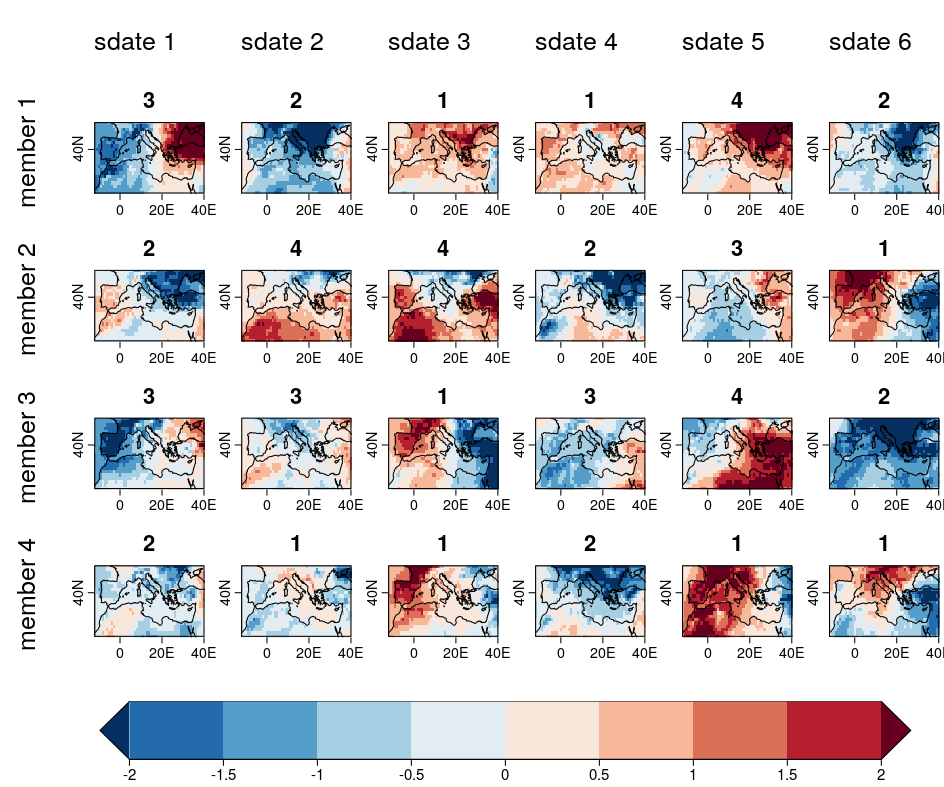## Ensemble clustering

### Introduction

Ensemble forecasts provide insight on average weather conditions from sub-seasonal to seasonal timescales. With large ensembles, it is useful to group members according to similar characteristics and to select the most representative member of each cluster. This allows to characterize forecast scenarios in a multi-model (or single model) ensemble prediction. In particular, at the regional level, this function can be used to identify the subset of ensemble members that best represent the full range of possible outcomes that may be fed in turn to downscaling applications. The choice of the ensemble members can be done by selecting different options within the function in order to meet the requirements of specific climate information products that can be tailored to different regions and user needs.

For a detailed description of the tool please refer to the CSTools guide : https://cran.r-project.org/package=CSTools

### Steps of the vignette

This vignette consists of three main steps: 1. Preliminary setup, 2. Loading the data, 3. Launching Ensemble clustering, 4. Results retrieval, 5. Results mapping, 6. Final notes.

### 1. Preliminary setup

To run this vignette, install and load Library CSTools

``````install.packages("CSTools")
library(CSTools)
``````

and the following packages:

``````install.packages("s2dv")
library(s2dv)
``````

For our example we will use the sample seasonal temperature data provided within the CSTools package. Data can be loaded as follows:

``````datalist <- CSTools::lonlat_data\$exp
``````

The data will has the following dimension:

``````dim(datalist\$data)
dataset  member   sdate   ftime     lat     lon
1      15       6       3      22      53
``````

Therefore the number of members is 15, the number of start dates is 6, while the forecast time steps are 3. The lat and lon dimensions refer to a 22x53 grid.

### 3. Launching Ensemble clustering

Prior to launching EnsClustering let's define the number of clusters, e.g., 4

``````numcl = 4
``````

Let's launch the clustering using 4 clusters (numclus), 4 EOFs (numpcs), 'mean' as moment to apply to the time dimension (time_moment), and both members and starting dates, i.e. 'c(“member”,“sdate”)', as dimension over which the clustering is performed (cluster_dim).

``````results <- CST_EnsClustering(datalist, numclus = numcl, numpcs = 4,
time_moment = 'mean', cluster_dim = c('member', 'sdate'))
``````

The EnsClustering produces the following outputs saved in object results:

``````names(results)
# "cluster"        "freq"           "closest_member" "repr_field"
# "composites"     "lat"            "on"

``````

where:

• \$cluster contains the cluster assigned for each 'member' and 'sdate'
• \$freq contains relative frequency by 'cluster'
• \$closest_member the representative member for each 'cluster'
• \$repr_field contains list of fields for each representative member 'cluster', 'lat' and 'lon'
• \$composites contains the list of mean fields for 'cluster', 'lat' and 'lon'
• \$lat contains the 22 grid point latitudes
• \$lon containing the 53 grid point longitudes

So, for instance, the overall frequency per cluster can be displayed by querying the '\$freq' in 'results' obtaining:

``````results\$freq

[,1]
[1,] 35.55556
[2,] 27.77778
[3,] 21.11111
[4,] 15.55556
``````

Further, the cluster number to which each 'member - start-date' pair is assigned can be displayed by quering '\$cluster' in 'results' as shown below (members (15) are in row and the start-dates (6) are in column (i.e. 15*6 pairs).

``````results\$cluster

[,1] [,2] [,3] [,4] [,5] [,6]
[1,]    3    2    1    1    4    2
[2,]    2    4    4    2    3    1
[3,]    3    3    1    3    4    2
[4,]    2    1    1    2    1    1
[5,]    4    4    1    3    3    1
[6,]    4    1    1    2    4    1
[7,]    1    2    1    2    2    1
[8,]    4    2    1    2    4    1
[9,]    3    3    1    1    4    3
[10,]    1    2    1    2    4    1
[11,]    3    3    2    3    3    1
[12,]    3    1    2    1    2    2
[13,]    2    1    1    3    3    1
[14,]    2    2    4    1    1    2
[15,]    3    4    1    2    3    2
``````

### 4. Results retrieval

Let's keep in mind that the aim is that of identifying 'members - start-date' pairs to be retained in order to reduce the ensemble while keeping a good representativeness. To achieve this we can get an idea of how the clustering has performed by looking at the average cluster spatial patterns, which are found in the 'composites' argument of 'results' and have the following dimensions (we will map them at point 5):

``````dim(results\$composites)
cluster     lat     lon dataset
4      22      53       1
``````

while the 'repr_field' argument of 'results' provides the spatial pattern of the member lying closes to the centroid (has the same dimensions as those of 'composites'):

``````dim(results\$repr_field)
cluster     lat     lon dataset
4      22      53       1
``````

Finally, the pairs 'member - start-dates' to be picked as representative for each cluster are found in the 'closest_member' argument.

``````results\$closest_member

\$member
[,1]
[1,]    6
[2,]    1
[3,]   11
[4,]    6

\$sdate
[,1]
[1,]    6
[2,]    6
[3,]    1
[4,]    5
``````

### 5. Results mapping

The following lines produce a multiplot of the representative temperature anomalies spatial pattern per cluster (Figure 1). These are actually the closest realizations (member - start-date pair) to the cluster centroids noted as 'repr_field' above.

``````EnsMean <- MeanDims(datalist \$data, c('member', 'sdate', 'ftime'))
EnsMean <- InsertDim(Reorder(EnsMean, c("lat", "lon", "dataset")),
posdim = 1, lendim = 4, name = 'cluster')

PlotLayout(PlotEquiMap, plot_dims = c("lat", "lon"),
var = results\$repr_field[,,,1] - EnsMean[,,,1],
lon = results\$lon, lat = results\$lat, filled.continents = FALSE,
titles = c("1","2","3","4"), brks = seq(-2, 2, 0.5),
fileout = "EnsClus_4clus_both_mem_std_Fig1.png")
``````{width=100%}

The lines below produce a multiplot of the temperature anomaly patterns for each 'member - start-date' pair and the respective cluster to which they are assigned (Figure 2). We limit the multiplot to the first 24 out of 90 combinations (i.e. 15 members*6 start_dates = 90) for figure clarity.

``````ExpMean <- MeanDims(datalist\$data, 'ftime')
EnsMean <- InsertDim(InsertDim(InsertDim(EnsMean[1,,,],
posdim = 1, lendim = 6, name = 'sdate'),
posdim = 1, lendim = 15, name = 'member'),
posdim = 1, lendim = 1, name = 'dataset')
ExpMeanSd <- Reorder(ExpMean - EnsMean, c('dataset', 'sdate', 'member' , 'lat', 'lon'))
PlotLayout(PlotEquiMap, plot_dims = c("lat", "lon"),
var = ExpMeanSd[, , 1:4, , ], title_scale = 0.7,
ncol = 6, nrow = 4, row_titles = paste('member' , 1:4), col_titles = paste('sdate', 1:6),
lon = results\$lon, lat = results\$lat, filled.continents = FALSE,
titles = as.character(t(results\$cluster[1:4, 1:6,])), brks = seq(-2, 2, 0.5),
width = 24, height = 20, size_units = 'cm',
fileout = "EnsClus_4clus_both_mem_std_Fig2.png")
``````{width=100%}

### Final notes

It should be noted that the clustering can be carried out with respect to 'members' or 'start-dates' alone. In which case the selection is no longer done over all 'member - start-dates' pairs (90) but along the 15 members (with all start-dates) or along the 6 start-dates (with all members). The clustering command with respect to 'members' is listed below:

``````print('clustering over members')
results_memb <- CST_EnsClustering(datalist, numclus = numcl, numpcs = 4, time_moment = 'mean', cluster_dim = 'member')
``````

Also, in addition to 'mean', the 'time_moment' can be set to 'sd', i.e. standard deviation along time, or 'perc', i.e. a percentile along time (in which case the 'time_percentile' argument is also needed). The latter option 'perc' may be useful when considering extremes, so for instance while analysing extreme temperatures with a high percentile (e.g. 90). The clustering command with respect to 'member - start-dates' pairs over the 90th percentile of temperature (i.e. high temperatures) is listed below:

``````print('clustering using time_percentile')
results_perc <- CST_EnsClustering(datalist, numclus = numcl, numpcs = 4, time_moment = 'perc', time_percentile = 90, cluster_dim = c('member', 'sdate'))
``````

Finally, the parameter 'time_dim' lets the user specify the dimension(s) over which the 'time_moment' is applied (i.e. mean, sd, perc). If 'time_dim' is not specified, the dimension is sought automatically picking the first between 'ftime', 'sdate', and 'time'.